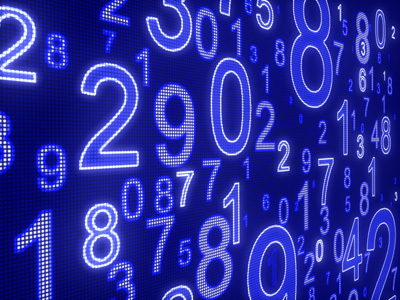Improve your Spanish math skills in this subtraction quiz.

# Math - Subtraction in Spanish

This is a continuation quiz in our Spanish Easy Review math series and looks at subtraction. In this quiz, rather than use numerical numbers to do math we will be using the written word for the number. Naturally those words will be in Spanish. If you have not already done so, you might want to take a look at the Spanish Easy Review math quiz titled Addition in Spanish as that will give you a good understanding of what you will be doing here. This quiz will only deal with subtracting numbers to see if you can find the correctly spelled out answer in Spanish. Let’s do two examples.

treinta y cinco - quince = veinte (35 - 15 = 20)

setenta y tres - treinta y uno = cuarenta y dos (73 - 31 = 42)

These math problems are not hard but they will make you have to think about the answers in Spanish. You may have already heard and read that part of learning another language is to learn to think in that language. Doing math is a great way to help accomplish that goal! So, if you are ready, then move on to the following ten Spanish math problems and see if you can quickly find the correct answer.

1.
veinte y seis – doce = ____
veinte y cuatro
quince
treinta y ocho
catorce
veinte y seis – doce = catorce (26 – 12 = 14).
2.
cuarenta y uno – diez y seis = ____
veinte y cinco
treinta y siete
cincuenta y siete
veinte y tres
cuarenta y uno – diez y seis = veinta y cinco (41 – 16 = 25).
3.
cincuenta y siete – cincuenta y siete = ____
uno
cero
seis
once
cincuenta y siete – cincuenta y siete = cero (57 – 57 = 0).
4.
treinta y ocho – diez = ____
diez y ocho
cuarenta y ocho
veinte y ocho
veinte y uno
treinta y ocho – diez = veinte y ocho (38 – 10 = 28).
5.
nueve – siete = ____
tres
dos
uno
cero
nueve – siete = dos (9 – 7 = 2).
6.
noventa y tres – cincuenta y siete = ____
veinte
cuarenta y tres
veinte y tres
treinta y seis
noventa y tres – cincuenta y siete = treinta y seis (93 – 57 = 36).
7.
diecinueve – catorce = ____
cuatro
quince
cinco
seis
diecinueve – catorce = cinco (19 – 14 = 5).
8.
setenta y ocho – sesenta = ____
dieciocho
dieciséis
diecinueve
diecisiete
setenta y ocho – sesenta = dieciocho (78 – 60 = 18).
9.
cien – sesenta y ocho = ____
cincuenta y dos
treinta y dos
cuarenta y dos
setenta y dos
cien – sesenta y ocho = treinta y dos (100 – 68 = 32).
10.
setenta y cuatro – veinte y seis = ____
cuarenta y ocho
cincuenta y uno
sesenta
treinta y nueve
setenta y cuatro – veinte y seis = cuarenta y ocho (74 – 26 = 48).
Author:  Christine G. Broome